8th Grade Math Home > Teacher Resources > Activities > A Human Number Line: Teacher Notes

A Human Number Line: Teacher Notes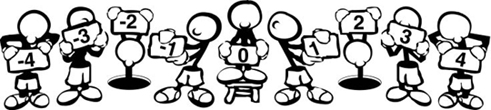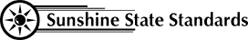MA.5.A.6.4
MA.7.A.3.1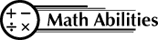Conceptual Knowledge

Number Line
Symbols
Equality
Inequality

Procedural Knowledge

Subtraction
Solving Equations and Inequalities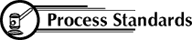Problem Solving
Reasoning
Communication
Connections
Representation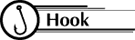Can we make a human number line?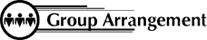Students work as a class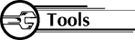21 pieces of tagboard approximately 6" by 12" to represent the integers from -10 to 10. Each piece of tagboard should have one integer written on it. Reverse side is identical with open dot.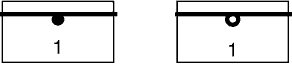Pass out the integers and line the students up to form a number line, zero in the middle, negative numbers to the left, and positive numbers to the right.

1. Strategy 1 Pictorial Representation:
Let P represent +1. Let N represent -1.
Reinforce the following concepts:
P and N are called a zero pair.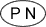= 0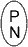= 0

When adding, represent both numbers and combine zero pairs, if possible. When subtracting, represent the first number and from that representation subtract the second number.

Examples:
1. 4 + (-3) = ?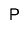= +1
2. -5 + (-2) = ?
N N N N N
N N
= -7
3. 4 - (-3) = ?
P P P P
You need to subtract 3 N's, but there are no N's.
Add zero pairs (P N) until you can subtract 3 N's.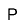Now subtract 3 N's and the result is P P P P P P P = +7
4. -4 - (-3) = ?
N N N N
You need to subtract 3 N's.
The result is N or -1.
5. -3 -(-5) =?
N N N
You need to subtract 5 N's, but there are not enough N's.
Add zero pairs (P N) until you can subtract 5 N's.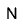Now subtract 5 N's and the result is P P = +2.

Once students have practiced with pictorial representations, how would you make connections to the abstract concepts and procedural skills of adding and subtracting integers?

For example,
 -3 - (-5) = 0 + 0 + (-3) - (-5) = (1 + -1) + (1 + -1) + (-3) - (-5) = (1 + 1) + (-1 + -1 + -3) - (-5) = +2 + (-5) - (-5) -3 - (-5) = +2

2. Strategy 2 Number Line:
Reinforce the following concepts related to the number line:
The first number in the expression is where you start on the number line.
Operation symbols for adding (+) and subtracting (-) are called the facing command. Addition means you face forward (right) and subtraction means you face to the back (left).
Symbols inside the parenthesis (or with the second number) are called the moving commands. The positive sign means to move forward, while the negative sign means to move backwards.

Examples:
1. 4 + (-3) = ?
Start at 4.
Face forward (to the right).
Move 3 spaces backwards.
End up at 1.
2. -5 + (-2) = ?
Start at -5.
Face forward (to the right).
Move 2 spaces backwards.
End up at -7.
3. 4 - (-3) = ?
Start at 4.
Face backward (to the left).
Move 3 spaces backwards.
End up at +7.
4. -4 - (-3) = ?
Start at -4.
Face backwards (to the left).
Move 3 spaces backwards.
End up at -1.
5. -3 - (-5) = ?
Start at -3.
Face backwards (to the left).
Move 5 spaces backwards.
End up at +2.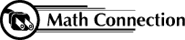As a result of this activity, students will have a better understanding of addition and subtraction of integers and the relative size of numbers.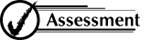• Have students on the number line show graphing for equations and inequalities.
• Have students at seats write equation or inequality after seeing those graphed.

 8th Grade Math Home > Teacher Resources > Activities > A Human Number Line: Teacher Notes